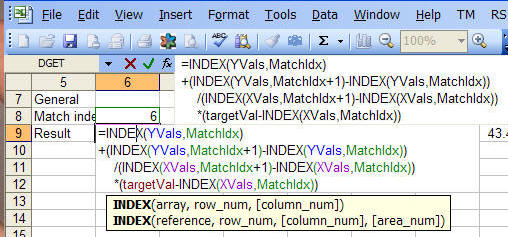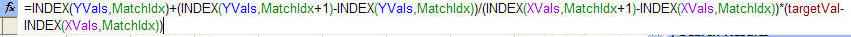You are on the Home/Excel/Tutorials/Format formulas pageShare Your

# Formatting a formula

A not so well documented feature of Excel is that it is possible to format a formula to improve readability.  For example, it is possible to have a formula look like:

```=INDEX(YVals,MatchIdx)
+(INDEX(YVals,MatchIdx+1)-INDEX(YVals,MatchIdx))
/(INDEX(XVals,MatchIdx+1)-INDEX(XVals,MatchIdx))
*(targetVal-INDEX(XVals,MatchIdx))```

with the corresponding screenshots

 "In-cell" editing:Editing in the formula barOf course, the typical and default formula would look like:

=INDEX(YVals,MatchIdx)+(INDEX(YVals,MatchIdx+1)-INDEX(YVals,MatchIdx))/(INDEX(XVals,MatchIdx+1)-INDEX(XVals,MatchIdx))*(targetVal-INDEX(XVals,MatchIdx))

or the corresponding screenshot:### How to format a formula

It's actually quite easy.  Use the key combination ALT+ENTER to create a 'new line' in a formula.  To add an indent simply use the space bar!

### A caveat

Under some circumstances that I cannot replicate, Excel will reformat the formula back to the default style.  So, please keep that in mind before you invest a significant amount of effort formatting formulas.